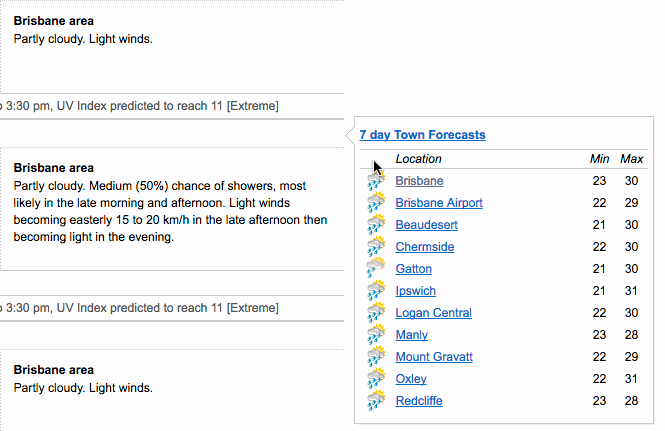datapasta

https://github.com/MilesMcBain/datapasta/stylerhttps://github.com/r-lib/styler

The goal of styler is to provide non-invasive pretty-printing of R source code while adhering to the tidyverse formatting rules. styler can be customized to format code according to other style guides too.

https://github.com/MilesMcBain/datapasta/
https://github.com/r-lib/styler
https://www.zhihu.com/question/398418315

Map NM ID to Gene Symbol

mysql --user=genome -N --host=genome-mysql.cse.ucsc.edu -A -D hg38 -e "select name,name2 from refGene"

library(plyranges)
gr <- read_gff("/path/to/gtf/or/gff") %>% select(transcript_id, gene_id, gene_name)
gr <- unique(data.frame(gr))

devtools::install_github("ProfessionalFarmer/loonR")
# 需要安装biomaRt和dplyr
mapping.table <- loonR::get_full_mapping_table()
# 保存mapping.table

#####################################################################
#版权所有 转载请告知 版权归作者所有 如有侵权 一经发现 必将追究其法律责任
#Author: Jason
#####################################################################

Fixation index (FST)

Subpopulation 1 Subpopulation 2 Subpopulation 3 Total
Genotype AA 125 50 100
Aa 250 30 500
aa 125 20 400
Number of individual 500 100 1000 1600
Number of alleles 1000 200 2000 3200
Step 1. Calculate the gene   (allele) frequencies
Observed allele frequency A (p) (125*2+250)/1000=0.5 (50*2+30)/200=0.65 (2*100+500)/2000=0.35
a (q) 0.5 0.35 0.65
Step 2. Calculate the expected   genotypic counts under Hardy-Weinberg Equilibrium, and then calculate the   excess or deficiency of homozygotes in each subpopulation.
Summary of homozygote deficiency or excess relative to HWE:
Pop. 1. Observed = Expected: perfect fit
Pop. 2. Excess of 15.5 homozygotes: some inbreeding
Pop. 3. Deficiency of 45 homozygotes: outbred or   experiencing a Wahlund effect (isolate breaking).
Expected allele frequency AA 500*0.5^2 = 125 (= observed) 100*0.65^2 = 42.25 (observed has excess of 7.75) 1,000*0.35^2 = 122.5   (observed has deficiency of 22.5)
Aa 500*2*0.5*0.5 = 250 (=   observed) 100*2*0.65*0.35 = 45.5 (observed has deficit of 15.5) 1,000*2*0.65*0.35 = 455   (observed has excess of 45)
aa 500*0.5^2 = 125 (= observed) 100*0.35^2 = 12.25 (observed has excess of 7.75) 1,000*0.35^2 = 422.5   (observed has deficiency of 22.5)
Step 3. Calculate the local   observed heterozygosities of each subpopulation (we will call them Hobs s,   where the s subscript refers to the sth of n populations — 3 in this   example).
Local observed   heterozygosities 250/500 = 0.5 (Hobs 1) 30/100 = 0.3 (Hobs 2) 500/1000 = 0.5(Hobs 3)
Step 4. Calculate the local   expected heterozygosity, or gene diversity, of each subpopulation
Hexp = 2pq
Local expected   heterozygosity 2*0.5*0.5=0.5 (Hexp 1) 2*0.65*3.5=0.455 (Hexp 2) 2*0.35*0.65=0.455 (Hexp 2)
Step 5. Calculate the local   inbreeding coefficient of each subpopulation
F = (Hexps -Hobs)/Hexp
[positive F means fewer heterozygotes than expected indicates   inbreeding]
[negative F means more heterozygotes   than expected means excess outbreeding]
F1=(0.5—0.5)/0.5=0 F2=(0.455—0.3)/0.455=0.341 F3=(0.455—0.5)/0.455=-0.099
Step 6. and 7. Calculate p-bar   (p-bar, the frequency of allele A) over the total population.
Calculate q-bar (q-bar, the frequency of allele a) over the total   population.
Check: p-bar + q-bar = 1.0
the frequency of allele over the total population p-bar (0.5*1000+0.65*200+0.35*2000)/3200=0.4156
q-bar (0.5*1000+0.35*200+0.65*2000)/3200=0.5844
Step 8. Calculate the global   heterozygosity indices (over Individuals, Subpopulations and Total   population)
HI based on observed heterozygosities in individuals in subpopulations
HS based on expected heterozygosities in subpopulations
HT based on expected heterozygosities for overall total population
HI (observed) (0.5*500+0.3*100+0.5*1000)/1600=0.4875
HS (expected) (0.5*500+0.455*100+0.455*1000)/1600=0.4691
HT (in overall total population) 2*p-bar *q-bar    = 2 * 0.4156 * 0.5844 = 0.4858
Step 9. Calculate the global   F-statistics
Compare and contrast the global FISbelow with the “local inbreeding   coefficient” Fs of Step 5.
Here we are using a weighted average of the individual heterozygosities   over all the subpopulations.
Both FIS and Fs are, however, based   on the observed heterozygosities,
whereas FST and FIT are based   on expected heterozygosities.
FIS (Hs-Hi)/Hs=(0.4691-0.4875)/0.4691=-0.0393
FST (Ht-Hs)/Ht=(0.4858-0.4691)/0.4858=-0.0344
FIT (Ht-Hi)/Ht=(0.4858-0.4875)/0.4858=-0.0036
Step 10 conclusions Finally, draw some   conclusions about the genetic structure of the population and its   subpopulations.
1) One of the possible HWE   conclusions we could make:
Pop. 1 is consistent with HWE (results of Step 2)
2) Two of the possible “local   inbreeding” conclusions we could make from Step 5:
Pop. 2 is inbred (results of Step 5), and
Pop. 3 may have disassortative mating or be experiencing a Wahlund effect   (more heterozygotes than expected).
3) Conclusion concerning overall   degree of genetic differentiation (FST)
Subdivision of populations, possibly due to genetic drift,
accounts for approx. 3.4% of the total genetic variation
(result of Eqn FST.8 FST   calculation in Step 9),
4) No excess or deficiency of   heterozygotes over the total population (FIT    is nearly zero).

Parse gtf

I always use gtf file and retrieve gene information. There isn’t a highly flexible tool to solve my demand. I modified the code from “https://github.com/Jverma/GFF-Parser”, thanks Jverma. This tool will be easier to use.Usage

Basically, there are three parameters.

id: either transcript id or gene id.

attType: attribute defined in gtf file. E.g. feature (column 3), gene_name, exon_number, transcript_id in column 9

attValue: the attribute value you want to search for.

>>> import sys
>>> from gtfParser import gtfParser

>>> gtf = gtfParser("example.gtf")

>>> # Get all exons in CDK7
>>> gtf.getRecordsByID("CDK7", "feature", "exon")

>>> # Get all features of transcript_id defined as "NM_001324069" in gene "CDK7"
>>> gtf.getRecordsByID("CDK7", "transcript_id", "NM_001324069")

>>> # Get start codon where feature was defined as "start_codon" in transcript "NM_001324069"
>>> gtf.getRecordsByID("NM_001324069", "feature", "start_codon")

>>> # Get a exon where its id is "NM_001324078.1" in "NM_001324078" transcript
>>> gtf.getRecordsByID("NM_001324078", "exon_id", "NM_001324078.1")

# Example gtf
Here is an simple example of gtf file. You can use to test. A subset from refSeq.hg38.gtf.

整合多组学数据的通路富集分析-ActivePathways

）中遇到的合并多个p-value的操作。这篇文章是今年发表在NC上。所有的组学或者大规模的数据分析，都需要探索数据背后相关的生物学功能，所以通路富集分析非常普遍。通常的做法是基于单一组学、单一数据集的数据进行分析，随着生物学数据的爆发，大规模多组学数据变得普遍，这篇文章介绍了基于整合的多组学或多数据集的数据进行通路分析的工具ActivePathways。方法

ActivePathways的方法，如下图：
(a) 需要的输入文件
(1) 基于多组学数据集的基因P-value，传统的富集分析是单组学，只有一列，现在是多组学，对应多列P-value
(2) 基因集，这个和其他的通路富集分析一样，用来表示生物学过程和通路

(b)
(1), 用Brown method合并基因的P-values，并且排序，用一个宽松的阈值来过滤检阳性的基因。
(2), 对每个通路，用排序的基因（从第一个开始从少到多作为sub-list）进行超几何检验，并找到最优的sub-list长度。
(3), 基因单一组学的数据进行富集分析，找到支持每个通路的证据。

(c) ActivePathways 提供整合之后的富集分析结果，相关的Brown P-value，支持通路的证据。还可以在Cytoscape中画Enrichmentmap的图，来分析更广泛的生物学主题。点为通路，边表示有共有基因。例子

(a) Enrichment map，其中蓝色的表示仅由整合数据发现的通路，比如凋亡等，显示出其强大的功能。
(b) 乳腺癌Basel和HER2亚型中，与预后相关的免疫基因的Hazard Ratio（HR），显示出两种亚型间不同的免疫pattern。
(c) HER2亚型中，与预后相关的基因（凋亡过程负调控）在每个数据集上的P-value和整合后的Brown P-value。其中DUSP1在三个数据集中都非常显著，如果作者聚焦到了这个基因。
(d) 这个基因在肿瘤细胞mRNA，癌旁细胞mRNA和拷贝数变异三种数据集中，分成high和low两组，做生存分析，可以发现DUSP1低表达，预后显著好于高表达，以此证明ActivePathway的强大。ActivePaths的下载地址：https://github.com/reimandlab/ActivePathways。为一个R包，整理好P-values的数据框之后，一步命令即可分析，此外结果还可以在Cytoscape上用Enrichment map展示。

# scores是P-values的数据框,GMT是基因集
ActivePathways(scores, fname_GMT)

参考

https://www.nature.com/articles/s41467-019-13983-9

#####################################################################
#版权所有 转载请告知 版权归作者所有 如有侵权 一经发现 必将追究其法律责任
#Author: Jason
#####################################################################